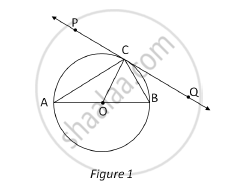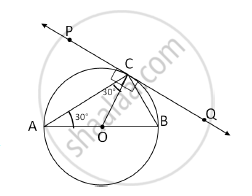Share

# In Fig. 1, PQ is a tangent at a point C to a circle with centre O. if AB is a diameter and ∠CAB = 30°, find ∠PCA. - CBSE Class 10 - Mathematics

#### Question

In Fig. 1, PQ is a tangent at a point C to a circle with centre O. if AB is a diameter and ∠CAB = 30°, find ∠PCA.#### Solution

In the given figure,
In ΔACO,

OA = OC …(Radii of the same circle)

∴ΔACO is an isosceles triangle.

∠CAB = 30° …(Given)

∴∠CAO = ∠ACO = 30°

…(angles opposite to equal sides of an isosceles triangle are equal)

∠PCO = 90° …(radius drawn at the point of contact is perpendicular to the tangent)

Now ∠PCA = ∠PCO – ∠CAO

∴∠PCA = 90° – 30° = 60°Is there an error in this question or solution?

#### Video TutorialsVIEW ALL 

Solution In Fig. 1, PQ is a tangent at a point C to a circle with centre O. if AB is a diameter and ∠CAB = 30°, find ∠PCA. Concept: Tangent to a Circle.
S